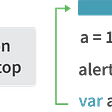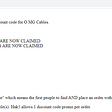# [Two Pointers] LC 986. Interval List Intersections

Goal

• Given two arrays, each contained close intervals, sorted in ascending order
• We are tasked to find the intersection of these close intervals

Example 1:

`Input: firstList = [[0,2],[5,10],[13,23],[24,25]], secondList = [[1,5],[8,12],[15,24],[25,26]]Output: [[1,2],[5,5],[8,10],[15,23],[24,24],[25,25]]`

Highlight

• Two pointers as the main technique
• Pointers are placed on each of the two arrays

Strategy

• As we mentioned above, pointers are placed on each of the two arrays
• We move each pointer forward only if we it is within another interval
• In each iteration, we use process two intervals by using max() and min() in order to figure out the intersection
• a = [1,5], b = [2,3] → [max(a, b), min(a, b)]
• And then we move the pointers

Complexity

• Time: O(N) as we traversed both arrays 1 time
• Space: O(N) as we created the output array

Code

`class Solution:    def intervalIntersection(self, firstList: List[List[int]], secondList: List[List[int]]) -> List[List[int]]:        l,r = 0, 0                res = []                while l < len(firstList) and r <len(secondList):            s = max(firstList[l], secondList[r])            e = min(firstList[l], secondList[r])            if s<=e:                res.append([s,e])                        if firstList[l] < secondList[r]:                l+=1            else:                r+=1                return res`

--

--

--

Data Engineering & Analytics

Love podcasts or audiobooks? Learn on the go with our new app.## Iris S

Data Engineering & Analytics

## More from Medium